5.3.2. Sphalerons in strong magnetic fields

The sphaleron, is a static and unstable solution of the field equations of the electroweak model, corresponding to the top of the energy barrier between two topologically distinct vacua . In the limit of vanishing Weinberg angle,w0, the sphaleron is a spherically symmetric, hedgehog-like configuration of SU(2) gauge and Higgs fields. No direct coupling of the sphaleron to a magnetic field is present in this case. Asw is turned on, the UY(1) field is excited and the spherical symmetry is reduced to an axial symmetry. A very good approximation to the exact solution is obtained using the Ansatz by Klinkhamer and Laterveer , which requires four scalar functions of r only,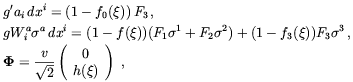(5.72)

where g and g' are the SU(2)L and U(1)Y gauge couplings, v is the Higgs VEV such that MW = g v / 2, Mh = sqrt[2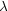] v,= gvr,a (a = 1, 2, 3) are the Pauli matrices, and the Fa's are 1-forms defined in Ref. . The boundary conditions for the four scalar functions are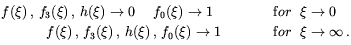(5.73)

It is known [230, 231] that forw0 the sphaleron has some interesting electromagnetic properties. In fact, differently from the pure SU(2) case, in the physical case a nonvanishing hypercharge current Ji comes-in. At the first order inw, Ji takes the form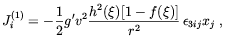(5.74)

where h and f are the solutions in thew0 limit, giving for the dipole moment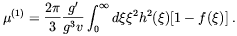(5.75)

The reader should note that the dipole moment is a true electromagnetic one because in the broken phase only the electromagnetic component of the hypercharge field survives at long distances.

Comelli et al.  considered what happens to the sphaleron when an external hypercharge magnetic field, BYext, is turned on. They found that the energy functional is modified as(5.76)

with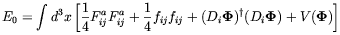(5.77)

and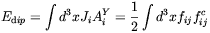(5.78)

with fiji AYj -j AYi. A constant external hypermagnetic field BextY directed along the x3 axis was assumed. In thew0 limit the sphaleron has no hypercharge contribution and then Edip(0) = 0 . At O(w), using (5.74) and (5.75) the authors of Ref.  got a simple magnetic dipole interaction energy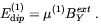(5.79)

In order to assess the range of validity of the approximation (5.79) one needs to go beyond the leading order inw and look for a nonlinear BextY-dependence of E. This requires to solve the full set of equations of motion for the gauge fields and the Higgs in the presence of the external magnetic field. Fortunately, a uniform BextY does not spoil the axial symmetry of the problem. Furthermore, the equation of motion are left unchanged (i fextij = 0) with respect to the free field case. The only modification induced by BextY resides in the boundary conditions since - as- we now have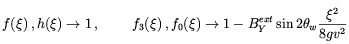(5.80)

whereas the boundary condition for0 are left unchanged.

The solution of the sphaleron equation of motions with the boundary conditions in the above were determined numerically by the authors of Ref. . They showed that in the considered BextY-range the corrections to the linear approximation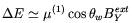are less than 5%. For larger values of BextY non-linear effects increase sharply. However, as we discussed in the previous sections, for such large magnetic fields the broken phase of the SM is believed to become unstable to the formation either of W-condensates  or of a mixed phase . In such situations the sphaleron solution does not exist any more. Therefore, it is safe to limit the previous analysis to values BextY0.4 T2.

The reduction of sphaleron energy due to the interaction with the field BcY has relevant consequences on the sphaleronic transition rate which is increased with respect to free field case. As a consequence, in an external magnetic field the relation between the Higgs VEV and the sphaleron energy is altered and Eq. (5.70) does not imply (5.69) any more. We can understand it by considering the linear approximation to E,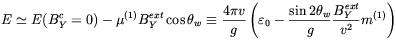(5.81)

where m(1) is the O(W) dipole moment expressed in units of e /W MW(T). From the Fig. 5.1 we see that even if v(Tc) / Tc1 the washout condition E / Tc35 is far from being fulfilled.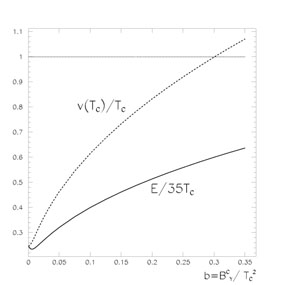Figure 5.1. The VEV at the critical temperature, v(Tc), and the sphaleron energy vs. the external magnetic field for Mh = MW. We see that even if v(Tc) / Tc1 the washout condition E / Tc35 is far from being fulfilled. From Ref. .

It follows form the previous considerations, that even if a strong magnetic fields might increase the strength of the EWPT, such an effect would not help baryogenesis.